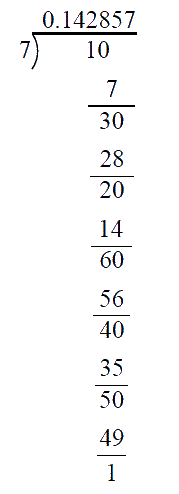# Ex.1.3 Q2 Number System Solution - NCERT Maths Class 9

## Question

You know that \begin{align}\frac{1}{7} = \overline {0.142857} .\end{align} Can you predict what the decimal expansions of \begin{align}\frac{2}{7},\frac{3}{7},\frac{4}{7},\frac{3}{7},\frac{4}{7},\frac{5}{7},\frac{6}{7}\end{align} are, without actually doing the long division? If so, how?

Video Solution
Number Systems
Ex 1.3 | Question 2

## Text Solution

What is Known?

The decimal expansion of \begin{align}\,\frac{1}{7}\end{align}.

What is Unknown?

The decimal expansions of \begin{align}\,\,\frac{2}{7},\frac{3}{7},\frac{4}{7},\frac{5}{7},\frac{6}{7}.\end{align}

Steps:

\begin{align}\frac{1}{7}=0 . \overline{142587}\end{align}This is a non-terminating recurring decimal.

We can use this to find the decimal expansion of \begin{align}\frac{2}{7},\,\,\frac{3}{7},\,\frac{4}{7},\,\frac{5}{7},\frac{6}{7}.\end{align}

To write the decimal expansion for:

i) \begin{align}\frac{2}{7}: \end{align} We observe that we get $$2$$ as remainder after the second step in the above division.

Hence,we start writing the quotient after the second decimal place and we get \begin{align}\frac{2}{7} = 0.\overline {285714} \end{align}

ii) \begin{align}\frac{3}{7}:\end{align} $$3$$ is the remainder after the first step.

\begin{align}\rm{Hence}\; \frac{3}{7}=0.\overline {428571}\end{align}

iii) \begin{align}\frac{4}{7}:\end{align} $$4$$ is the remainder at the $$4^{th}$$ step.

\begin{align}\text{Hence }\frac{4}{7}=0.\overline {571428} \end{align}

iv) \begin{align}\frac{5}{7}:\end{align}$$5$$ is the remainder at the $$5^{th}$$ step

\begin{align}\text{Hence }\frac{5}{7} = 0.\overline {714285} \end{align}

v)\begin{align}\frac{6}{7}:\end{align} $$6$$ is the remainder after the $$3^{rd}$$ step.

\begin{align}\text{Hence }\frac{6}{7} = 0.\overline {857142} \end{align}

Video Solution
Number Systems
Ex 1.3 | Question 2
Learn from the best math teachers and top your exams

• Live one on one classroom and doubt clearing
• Practice worksheets in and after class for conceptual clarity
• Personalized curriculum to keep up with school